# Rational Numbers Class 7 Notes: Chapter 9

According to the CBSE Syllabus 2023-24, this chapter has been renumbered as Chapter 8.

## Introduction to Rational Numbers

#### For more information on Types of Numbers, watch the below video.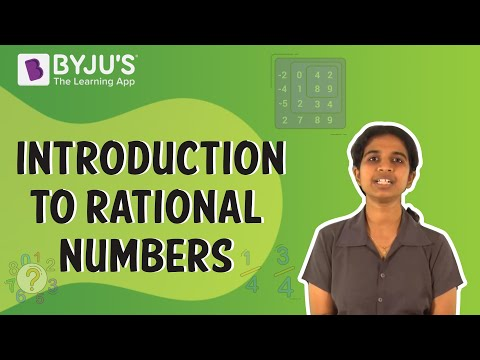### Introduction: Rational Numbers

• A rational number is defined as a number that can be expressed in the form
$$\begin{array}{l}\frac{p}{q}\end{array}$$
, where p and q are integers and q≠0.
• In our daily lives, we use some quantities which are not whole numbers but can be expressed in the form of
$$\begin{array}{l}\frac{p}{q}\end{array}$$
. Hence we need rational numbers.

### Equivalent Rational Numbers

• By multiplying or dividing the numerator and denominator of a rational number by a same non zero integer, we obtain another rational number equivalent to the given rational number. These are called equivalent fractions.
• $$\begin{array}{l}\frac{1}{3}=\frac{1}{3}\times \frac{2}{2}=\frac{2}{6}\end{array}$$

$$\begin{array}{l}\frac{2}{6}\end{array}$$
and
$$\begin{array}{l}\frac{1}{3}\end{array}$$
are equivalent fractions.
• $$\begin{array}{l}\frac{15}{25}=\frac{15\div5}{25\div5}=\frac{3}{5}\end{array}$$
$$\begin{array}{l}\frac{15}{25}\end{array}$$
and
$$\begin{array}{l}\frac{3}{5}\end{array}$$
are equivalent fractions.

### Rational Numbers in Standard Form

• A rational number is said to be in the standard form if its denominator is a positive integer and the numerator and denominator have no common factor other than 1.
• Example: Reduce
$$\begin{array}{l}\frac{-4}{16}\end{array}$$
.Here, the H.C.F. of 4 and 16 is 4.
$$\begin{array}{l}\Rightarrow \frac{-4}{16}=\frac{\frac{-4}{4}}{\frac{16}{4}}=\frac{-4}{16}\end{array}$$
$$\begin{array}{l}\frac{-1}{4}\end{array}$$
is the standard form of
$$\begin{array}{l}\frac{-4}{16}\end{array}$$
.

To know more about Rational Numbers, visit here.

### LCM

• The least common multiple (LCM) of two numbers is the smallest number (≠0) that is a multiple of both.
• Example: LCM of 3 and 4 can be calculated as shown below:
Multiples of 3: 0, 3, 6, 9, 12,15
Multiples of 4: 0, 4, 8, 12, 16
LCM of 3 and 4 is 12.

## Rational Numbers Between 2 Rational Numbers

### Rational Numbers between Two Rational Numbers

• There are unlimited number(infinite number) of rational numbers between any two rational numbers.
• Example: List some of the rational numbers between −35 and −13.
Solution: L.C.M. of 5 and 3 is 15.
⇒ The given equations can be written as
$$\begin{array}{l}\frac{-9}{15}\end{array}$$
and
$$\begin{array}{l}\frac{-5}{15}\end{array}$$
.
⇒ −615,−715,−815 are the rational numbers between −35 and −13.

Note: These are only few of the rational numbers between −35 and −13. There are infinite number of rational numbers between them. Following the same procedure, many more rational numbers can be inserted between them.

To know more about Rational Numbers Between 2 Rational Numbers, visit here.

## Properties of Rational Numbers

### Properties of Rational Numbers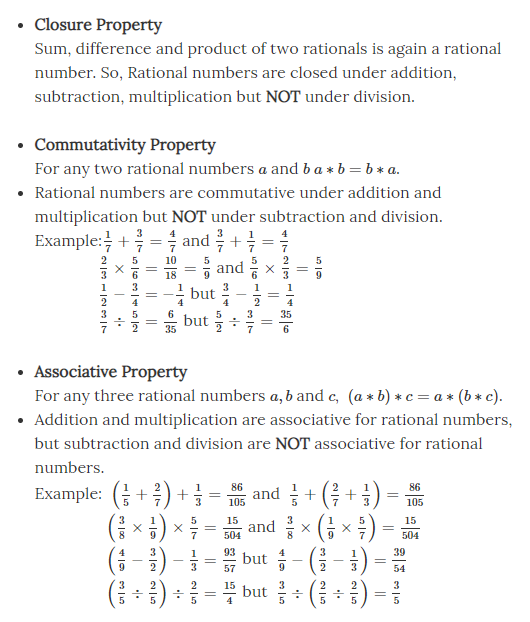To know more about Properties of Rational Numbers, visit here.

<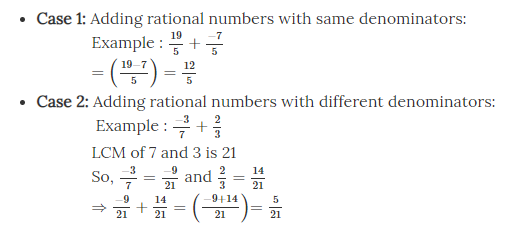### Subtraction of Rational Numbers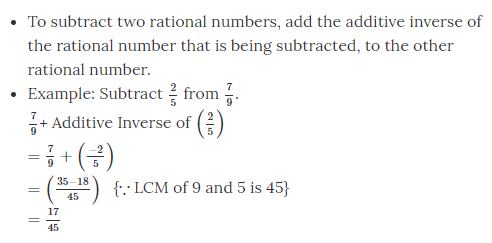### Multiplication and Division of Rational Numbers

Multiplication of Rational Numbers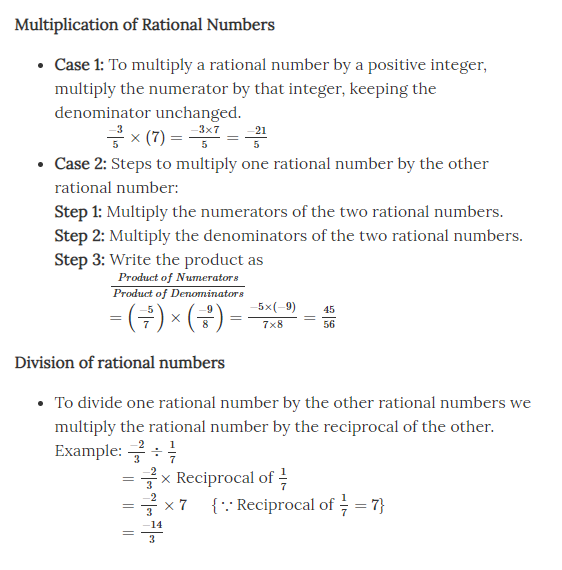To know more about Arithmetic Operations on Rational Numbers, visit here.

## Negatives and Reciprocals

### Negatives and Reciprocals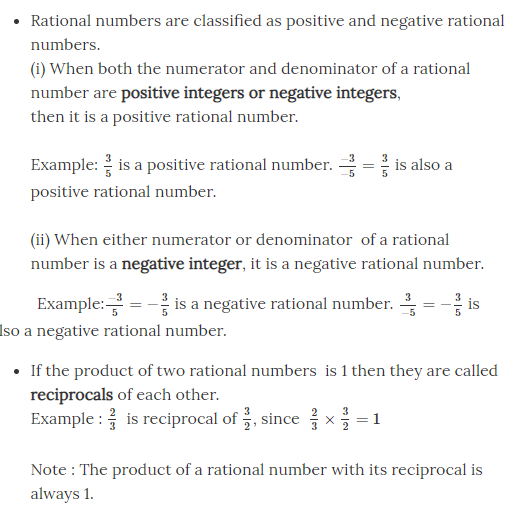### Additive Inverse of a Rational Number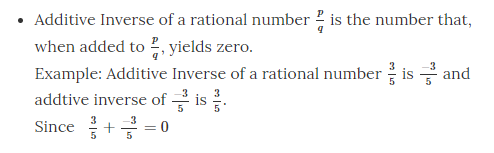## Representing on a Number Line

### Rational Numbers on a Number Line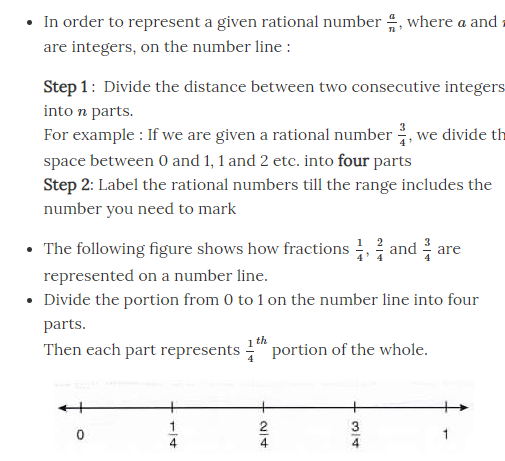To know more about Representation of a Rational Numbers on a Number Line, visit here.

### Comparison of Rational Numbers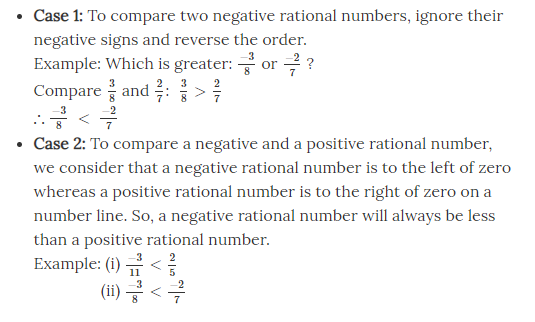## Frequently Asked Questions on CBSE Class 7 Maths Notes Chapter 9 Rational Numbers

Q1

### What are rational numbers?

A number that can be represented as the quotient p/q of two integers such that q ≠ 0 is called as a rational number.

Q2

### What are properties of rational numbers?

1. Closure property 2. Commutative property 3. Associative property 4. Distributive property 5. Identity property 6. Inverse property

Q3

### What is remainder theorem?

The remainder theorem states that when a polynomial, f(x), is divided by a linear polynomial , x – a, the remainder of that division will be equivalent to f(a).